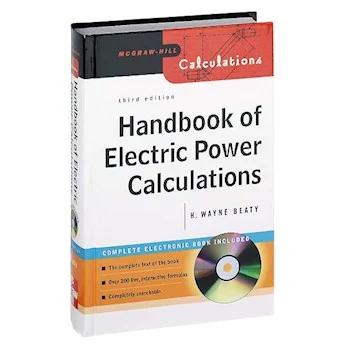# Handbook of Electric Power Calculations

## Handbook of Electric Power Calculations

H. Wayne Beaty

Preference :

The Handbook of Electric Power Calculations provides detailed step-by-step calculation
procedures commonly encountered in electrical engineering. The Handbook contains a
wide array of topics and each topic is written by an authority on the subject. The treatment
throughout the Handbook is practical with very little emphasis on theory.
Each of the 20 Sections follows this format:
• Clear statement of the problem.
• Step-by-step calculation procedure.
• Inclusion of suitable graphs and illustrations to clarify the procedure.
• Use of SI and USCS equivalents.

This relatively simple, yet comprehensive format adds greatly to the use of the Handbook
by the engineer or technician. Arithmetic and algebra are employed in the solution
of the majority of the problems. Each section contains a list of references or a bibliography
that is pertinent to the subject matter.Handbook of Electric Power Calculations

Content :
• Section 1. Basic Network Analysis
• Section 2. Instrumentation
• Section 3. dc Motors and Generators
• Section 4. Transformers
• Section 5. Three-Phase Induction Motors
• Section 6. Single-Phase Motors
• Section 7. Synchronous Machines
• Section 8. Generation of Electric Power
• Section 9. Overhead Transmission Lines and Underground Cables
• Section 10. Electric-Power Networks
• Section 11. Load-Flow Analysis in Power Systems
• Section 12. Power-Systems Control
• Section 13. Short-Circuit Computations
• Section 14. System Grounding
• Section 15. Power-System Protection
• Section 16. Power System Stability
• Section 17. Cogeneration
• Section 18. Stationary Batteries
• Section 19. Electric Energy Economic Methods
• Section 20. Lighting Design